# Math 753/853 HW4 Polynomial Interpolation¶

## Problem 1¶

(a) Write a function polyeval(c,x) that implements Horner's method for polynomial interpolation without base points. I.e. given a vector of monomial coefficients $c$ you want to evaluate the polynomial

\begin{equation*} P(x) = \sum_{n=0}^{m-1} c_n x^n \end{equation*}

using Horner's method of nested multiplication. E.g. write the function so that it evaluates the polynomial

\begin{equation*} P(x) = c_0 + c_1 x + c_2 x^2 + c_3 x^3 + c_4 x^4 \end{equation*}

with this series of multiplications and additions

\begin{equation*} P(x) = c_0 + x \, [c_1 + x \, [c_2 + x \, [c_3 + x \, c_4]]] \end{equation*}

Your function should have inputs $c$ and $x$, and it should return $P(x)$. Make sure the function works when $x$ is a vector, returning a vector of values $P(x)$. Your function should also work equally well on all numerical types.

Warning: Note that the natural mathematical expressions for polynomials have indices that start at 0, but that Julia and many other programming languages use indices that start at 1. You'll have to negotiate this difference when writing your Horner evaluation function

In :
# Evaluate polynomial p(x) = sum_n c_n x^n using Horner's method
function polyeval(c,x)
N = length(c);
p = c[N]
for n = N-1:-1:1
p = c[n] + x.*p
end
p
end

Out:
polyeval (generic function with 1 method)

(b) Test your polyeval(c,x) function on a simple quadratic or cubic polynomial of your choice. Do this by constructing a simple polynomial function in Julia, e.g. $f(x) = 1 + 2x - 3x^2$, plotting a few points (x, f(x)) with dots, and then plotting the same polynomial as a smooth line using your polyeval function with inputs c=[1 2 -3] and an x vector created with linspace. Label the axes and add a legend that shows which symbol is for the datapoints and which is for polyeval.

In :
using PyPlot
f(x) = 1 + 2x - 3x.^2
x = [-1, 0, 1]
plot(x,f(x), "bo", label="direct evaluation")

c = [1, 2, -3]
x = linspace(-1.1,1.1)
plot(x, polyeval(c,x), "r-", label="polyeval function")
xlabel("x")
ylabel("f(x) = 1 + 2x - 3x^2")
legend(loc="lower right")
grid("on")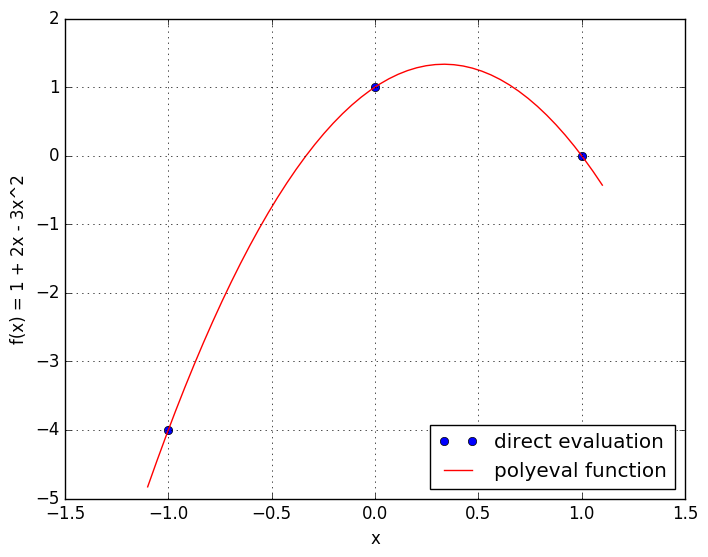## Problem 2¶

(a). Write a function polyeval(c,b,x) that implements Horner's method for polynomial interpolation with base points. E.g. given a vector of five coefficients c and four base points b you want to evaluate the polynomial

\begin{equation*} P(x) = c_0 + (x - b_0) \, [c_1 + (x - b_1) [c_2 + (x - b_2) [c_3 + (x - b_3) \, c_4]]] \end{equation*}

Your function should have inputs c, b, and x, and as in problem 1(a), it should work on vectors x of arbitrary numeric type.

In :
# Evaluate polynomial p(x) = sum_n c_n (x - b_n)^n using Horner's method
function polyeval(c,b,x)
N = length(c);
if length(b) ≠ N-1
println("horner(c,b,x) error: length(b) ≠ length(c)-1")
return zeros(x)
end
p = c[N]
for n = N-1:-1:1
p = c[n] + (x-b[n]).*p
end
p
end

Out:
polyeval (generic function with 2 methods)

(b) As in problem 1(b), test your polyeval(c,b,x) function on a simple polynomial graphically.

In :
# I'll test on
# P(x) = 4 + (x-1) [2 + (x+1) 3] = -1 + 2x + 3x^2
f(x) = -1 + 2x + 3*x.^2
x = [-1, 0, 1]
plot(x, f(x), "bo", label="explicit formula")

c = [4, 2, 3]
b = [1, -1]
x = linspace(-1.1,1.1)
plot(x, polyeval(c,b, x), "r-", label="polyeval function")
xlabel("x")
ylabel("f(x) = 1 + 2x - 3x^2")
legend(loc="upper left")
grid("on")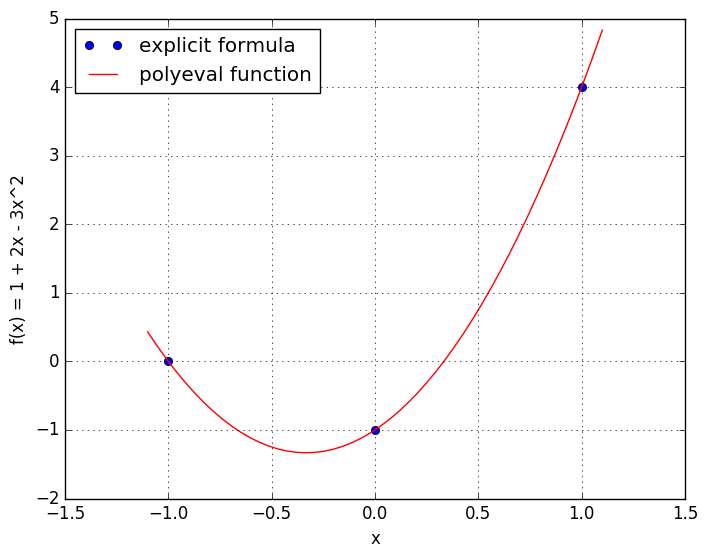WARNING: Method definition f(Any) in module Main at In:2 overwritten at In:3.


# Problem 3¶

(a) To familiarize yourself with the Newton Divided Differences algorithm, work out on paper (or in text/markdown in this notebook) the cubic interpolating polynomial for the $(x,y)$ data points $(-2,8), (0, 4), (1,2), (3,-3)$.

In :
# My calculation of the interpolating polynomial via Newton Divded Differences

#  xᵢ  f[xᵢ]
#
# -2   8
#            -2
#  0   4              0
#            -2             -1/30
#  1   2             -1/6
#            -5/2
#  3  -3
#
# So interpolating polynomial is
# P(x) = 8 + (x+2) [-2 + (x-0) [0 + (x-1) (-1/30)]]


(b) Make a plot that verifies your interpolating polynomial graphically, showing the data points as dots and the interpolant as a smooth curve.

In :
using PyPlot

xdata = [-2, 0, 1, 3]
ydata = [8, 4, 2, -3]
x = linspace(-2.1,3.1)
plot(xdata, ydata, "bo", label="data points")

x = linspace(-2.1,3.1)
P(x) = 8 + -2*(x+2) -1/30*(x+2).*x.*(x-1)
plot(x,P(x), "b--", label="explicit formula")
xlabel("x")
ylabel("y")
grid("on")
legend()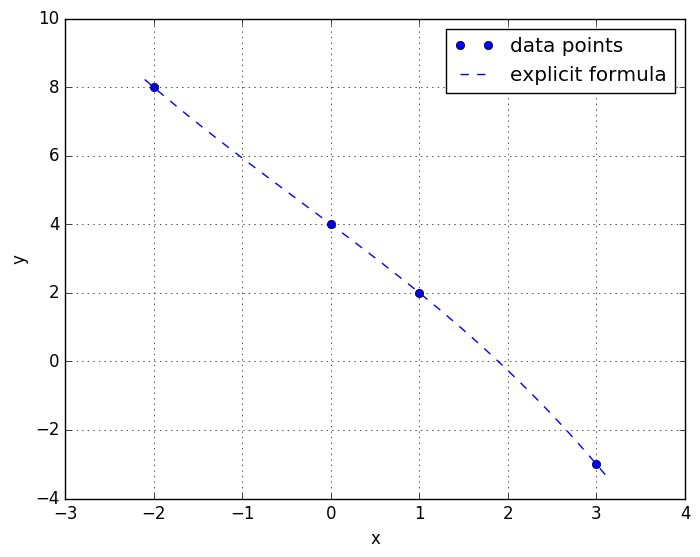Out:
PyObject <matplotlib.legend.Legend object at 0x7f7de999f150>

# Problem 4¶

(a) Write a function newtondivdiff(x,y) that returns (c,b), the polynomial coefficients c and the base points b for the polynomial interpolant that passes through the data points $(x_1, y_1), (x_2, y_2), \dots, (x_m, y_m)$. The return values $c$ and $b$ should be arranged to pass directly into your polyeval(c,b) function from problem 2.

In :
function newtondivdiff(x,y)
N = length(x);
if length(y) != N
println("newtondivdiff(x,y) error: length(x) ≠ length(y)")
return zeros(x)
end

F = zeros(N,N) # matrix to store divided differences
F[:,1] = y

for j=2:N
for i=1:N+1-j
F[i,j] = (F[i+1,j-1]-F[i,j-1])/(x[i+j-1]-x[i])
end
end
(F[1,:], x[1:N-1])
end

WARNING: Method definition newtondivdiff(Any, Any) in module Main at In:2 overwritten at In:2.

Out:
newtondivdiff (generic function with 1 method)

(b) Test your newtondivdiff(x,y) function with this series of steps

1. construct a quadratic or cubic polynomial, e.g. $f(x) = 1 + 2x - 3x^2$
2. construct a vector xdata with three values for a quadratic, or four for a cubic, e.g. xdata = [-2 -1 1].
3. evaluate $f$ at xdata to get a vector of $y$ values ydata
4. compute the coefficients c and basepoints b of the polynomial interpolant using newtondivdiff(xdata, ydata)
5. evaluate your polynomial interpolant on a large number of $x$ points, e.g. x = linspace(-2, 2); y = polyeval(c,b,x)
6. make a plot showing the datapoints xdata, ydata with dots and the smooth curve x,y with a line.
7. label the axes and provide a legend
In :
f(x) = 1 + 2x - 3x.^2 + 4x.^3
xdata = [-1, 0, 1, 2]
ydata = f(xdata)
plot(xdata, ydata, "bo", label="datapoints")

c,b = newtondivdiff(xdata, ydata)
x = linspace(-1.1,2.1)
plot(x, polyeval(c,b, x), "r-", label="interpolating polynomial")
xlabel("x")
ylabel("f(x) = 1 + 2x - 3x^2 + 4x^3")
legend(loc="upper left")
grid("on")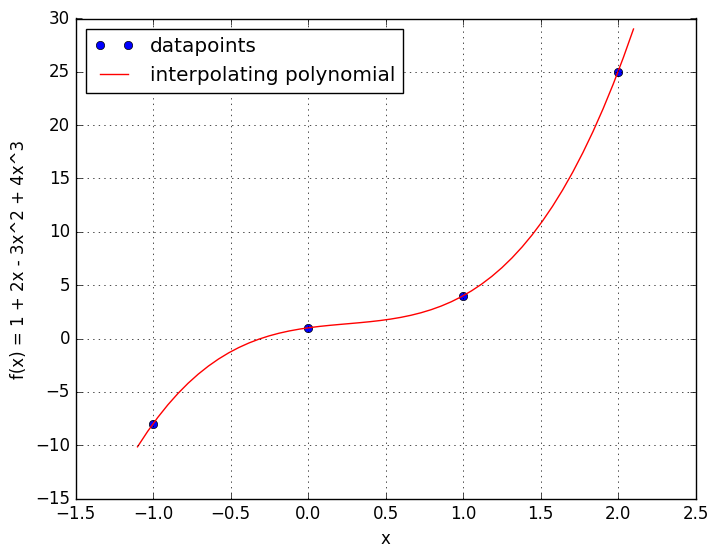WARNING: Method definition f(Any) in module Main at In:1 overwritten at In:1.


## Problem 5¶

The expected lifetime of an industrial fan decreases with operating temperature, according to the experimental data in this table

\begin{array}{c|c} temp~F & weeks \\ 77 & 57 \\ 104 & 45 \\ 122 & 38 \\ 140 & 32 \end{array}

Estimate the expected fan lifetime at 160 degrees Farenheit using polynomial interpolation. Make a plot showing the datapoints with dots and the interpolant over the range 50 to 160 weeks.

In :
xdata = [77, 104, 122, 140]
ydata = [57, 45, 38, 32]
c,b = newtondivdiff(xdata, ydata)

plot(xdata, ydata, "bo", label="experimental data points")
x=linspace(50,160)
y=polyeval(c,b,x)
plot(x, y, "r-", label="polynomial interpolant")
xlabel("temp F")
grid("on")
legend()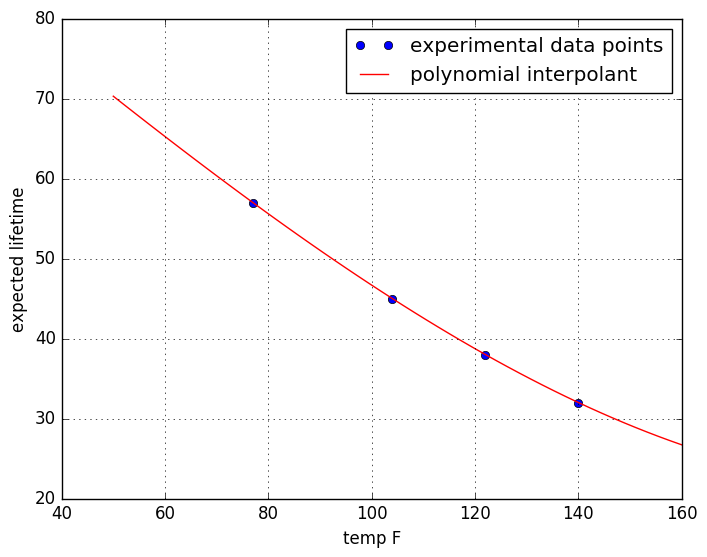Out:
PyObject <matplotlib.legend.Legend object at 0x7f7de6060ad0>
In :
# The expected lifetime at 160F will be this many weeks
polyeval(c,b,160)

Out:
26.714677640603572

## Problem 6¶

(a) Given these estimates of world human population over the last fifty years

\begin{array}{c|l} year & population~(billions) \\ 1960 & 3.026 \\ 1970 & 3.691 \\ 1980 & 4.449 \\ 1990 & 5.321 \\ 2000 & 6.128 \\ 2010 & 6.916 \end{array}

estimate the world population in 2025 by extrapolating the polynomial interpolant.

In :
tdata1 = [1960, 1970, 1980, 1990, 2000, 2010]
ydata1 = [3.026, 3.691, 4.449, 5.321, 6.128, 6.916]
c1,b1 = newtondivdiff(tdata1, ydata1)

# The estimated world population in 2025, is (in billions)
polyeval(c1,b1, 2025)

Out:
9.86719921874999

(b) Add the current estimated world population of 7.404 billion in 2016 to the data set and give a revised estimate of 2025 population.

In :
tdata2 = [1960, 1970, 1980, 1990, 2000, 2010, 2016]
ydata2 = [3.026, 3.691, 4.449, 5.321, 6.128, 6.916, 7.404]
c2,b2 = newtondivdiff(tdata2, ydata2)

# The estimated world population in 2025, is (in billions)
polyeval(c2,b2, 2025)

Out:
7.810654127038043

(c) Make a plot showing the datapoints and the two polynomial interpolants over the range 1950 to 2030. Plot the interpolant without the 2016 data in blue and with the 2016 data in red.

In :
plot(tdata2, ydata2, "bo", label="historical data points")
t=linspace(1950,2030)
plot(t, polyeval(c1,b1,t), "r-", label="polyinterp without 2016 data")
plot(t, polyeval(c2,b2,t), "m-", label="polyinterp with 2016 data")
xlabel("t")
ylabel("world population (billions)")
legend(loc="upper left")
grid("on")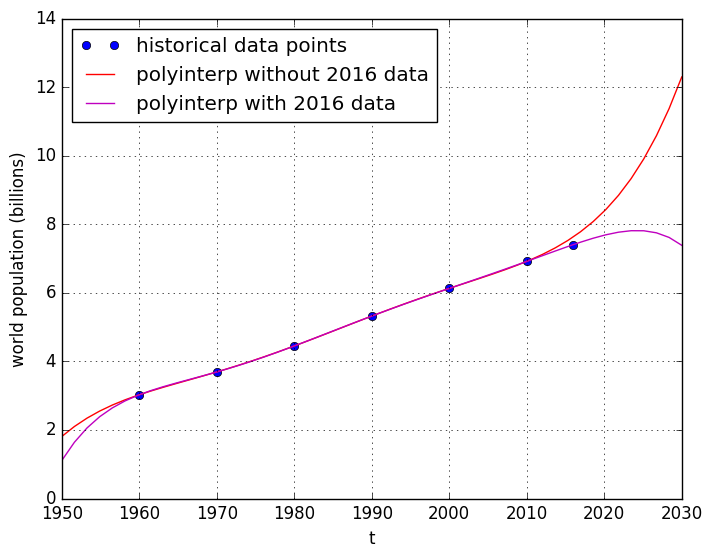(d) What conclusions do you draw from the difference between the two curves?

In :
# Extrapolation of interpolating polynomials seems pretty sensitive! Adding just one
# data point at 2016 changes the extrapolated value at 2025 from 9.8 billion down to
# 7.8 billion, about a 20% difference. Maybe this data would be better fit by an
# exponential, or by more data points and a least-squares, lower-order polynomial.

In [ ]: